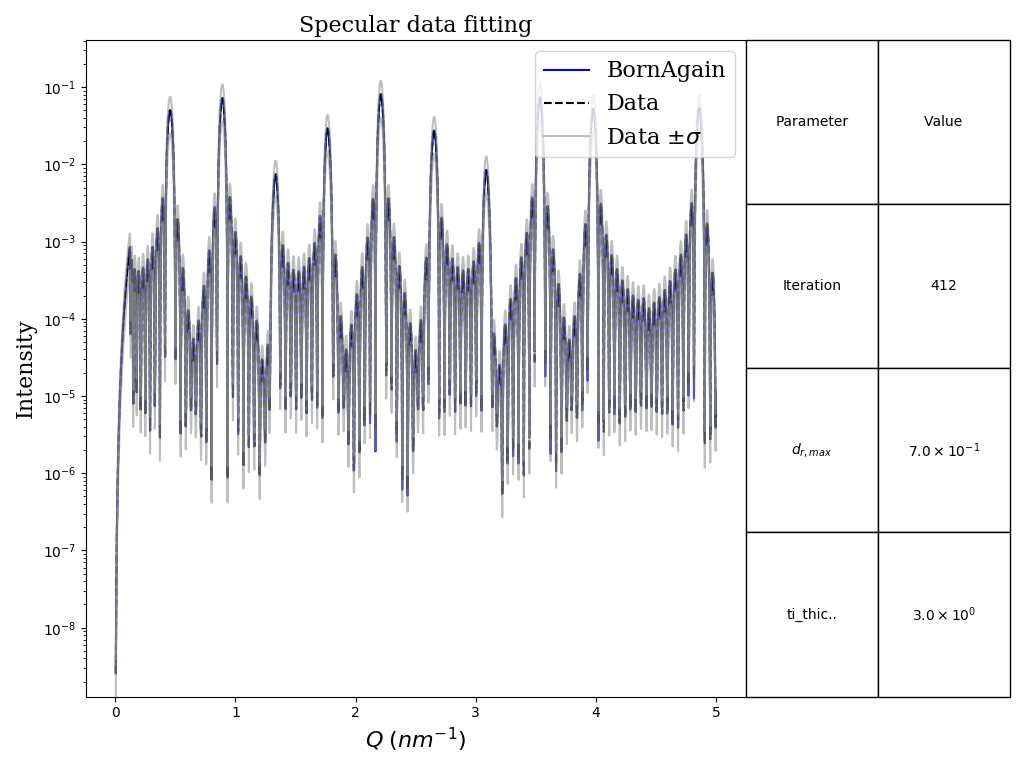## Taking uncertainties into account

In this example we are demonstrating how to allow for uncertainties during a Reflectometry fitting job. The sample to fit consists of twenty Titanium-Nickel bilayers. Assuming that all Titanium layers have the same thickness, the goal is to find that thickness.

The reference data was generated with GENX, setting the thickness of the Ti layers equal to 3 nm.

The main points to focus on here are the following:

• Added artificial uncertainties to the data being fitted
• Use of the the $RQ^4$ view for plotting
• Use of $\chi^2$ with $L_1$ normalization as the objective metric
• Setting a genetic algorithm as the minimizerFitting with uncertainties plot. Notice the $RQ^4$ scale of the Intensity axis
  1 2 3 4 5 6 7 8 9 10 11 12 13 14 15 16 17 18 19 20 21 22 23 24 25 26 27 28 29 30 31 32 33 34 35 36 37 38 39 40 41 42 43 44 45 46 47 48 49 50 51 52 53 54 55 56 57 58 59 60 61  #!/usr/bin/env python3 """ Example demonstrates how to fit specular data. Our sample represents twenty interchanging layers of Ti and Ni. We will fit thicknesses of all Ti layers, assuming them being equal. Reference data was generated with GENX for ti layers' thicknesses equal to 3 nm This example uses exactly the same data as in FitSpecularBasics. However this time we will add artificial uncertainties and use RQ^4 view. Besides we will set Chi squared with L1-normalization as the objective metric and use genetic algorithm as the minimizer. """ import os import numpy as np from matplotlib import pyplot as plt import bornagain as ba from bornagain import ba_fitmonitor import FitSpecularBasics as fsb def run_fitting(): """ Setup simulation and fit """ real_data = fsb.get_real_data_values() # setting artificial uncertainties (uncertainty sigma equals a half # of experimental data value) uncertainties = real_data*0.5 fit_objective = ba.FitObjective() fit_objective.addSimulationAndData(fsb.get_simulation, real_data, uncertainties) plot_observer = ba_fitmonitor.PlotterSpecular(units=ba.Coords_RQ4) fit_objective.initPrint(10) fit_objective.initPlot(10, plot_observer) fit_objective.setObjectiveMetric("Chi2", "L1") params = ba.Parameters() params.add("ti_thickness", 50*ba.angstrom, min=10*ba.angstrom, max=60*ba.angstrom) minimizer = ba.Minimizer() minimizer.setMinimizer("Genetic", "", "MaxIterations=40;PopSize=10") result = minimizer.minimize(fit_objective.evaluate, params) fit_objective.finalize(result) if __name__ == '__main__': datadir = os.getenv('BA_EXAMPLE_DATA_DIR', '') data_fname = os.path.join(datadir, "genx_interchanging_layers.dat.gz") fsb.get_real_data(data_fname) run_fitting() plt.show() 
auto/Examples/fit/specular/FitWithUncertainties.py

Data to be fitted: Examples/data/genx_interchanging_layers.dat.gz